Anzeige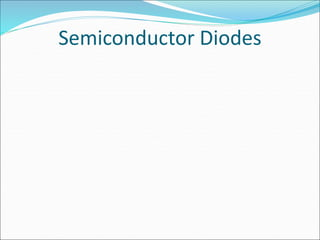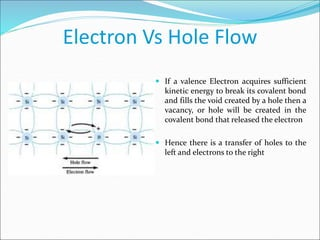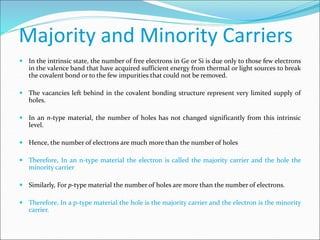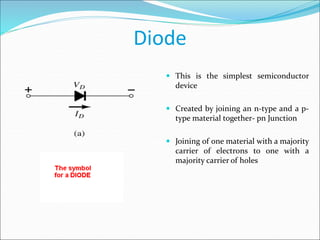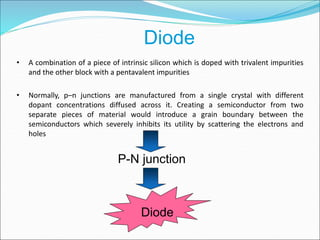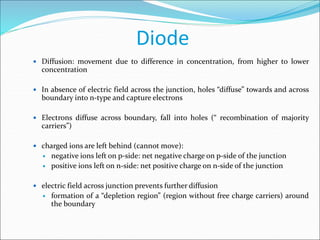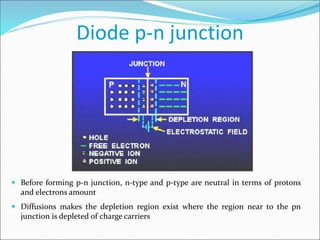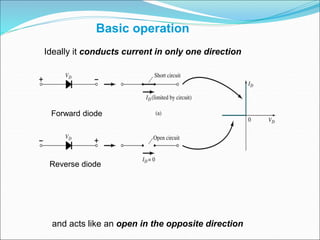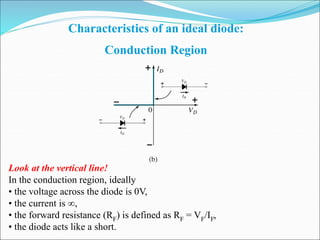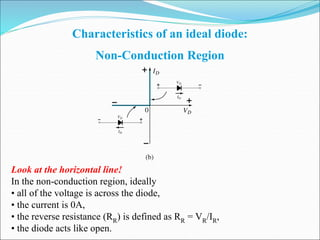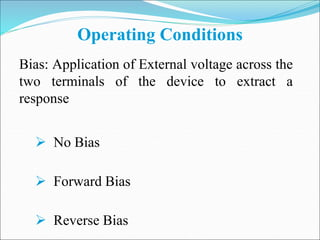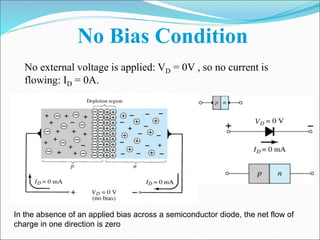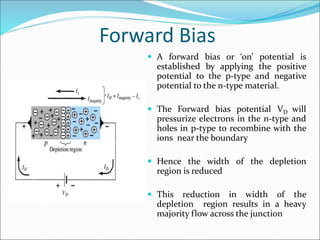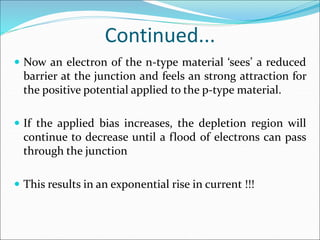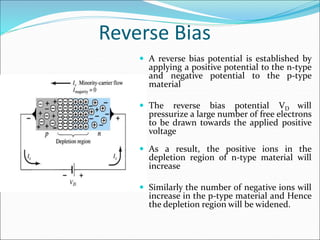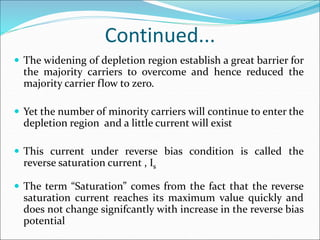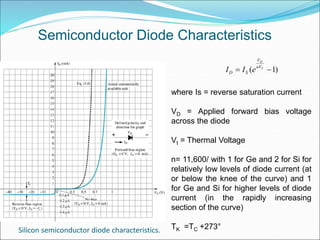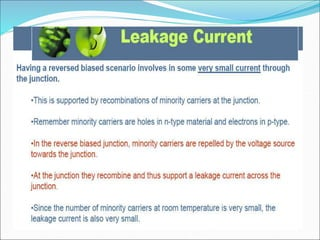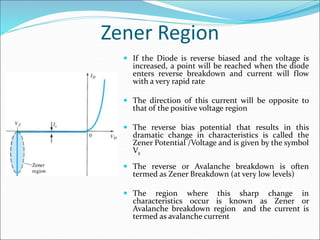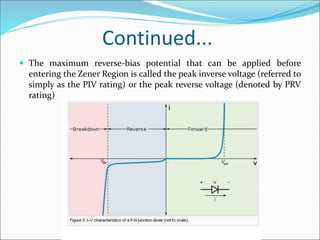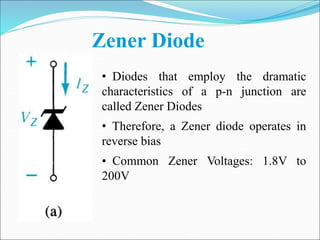1 von 21
Anzeige

### Semiconductor diodes1

1. Semiconductor Diodes
2. Electron Vs Hole Flow  If a valence Electron acquires sufficient kinetic energy to break its covalent bond and fills the void created by a hole then a vacancy, or hole will be created in the covalent bond that released the electron  Hence there is a transfer of holes to the left and electrons to the right
3. Majority and Minority Carriers  In the intrinsic state, the number of free electrons in Ge or Si is due only to those few electrons in the valence band that have acquired sufficient energy from thermal or light sources to break the covalent bond or to the few impurities that could not be removed.  The vacancies left behind in the covalent bonding structure represent very limited supply of holes.  In an n-type material, the number of holes has not changed significantly from this intrinsic level.  Hence, the number of electrons are much more than the number of holes  Therefore, In an n-type material the electron is called the majority carrier and the hole the minority carrier  Similarly, For p-type material the number of holes are more than the number of electrons.  Therefore, In a p-type material the hole is the majority carrier and the electron is the minority carrier.
4. Diode  This is the simplest semiconductor device  Created by joining an n-type and a p- type material together- pn Junction  Joining of one material with a majority carrier of electrons to one with a majority carrier of holes
5. Diode • A combination of a piece of intrinsic silicon which is doped with trivalent impurities and the other block with a pentavalent impurities • Normally, p–n junctions are manufactured from a single crystal with different dopant concentrations diffused across it. Creating a semiconductor from two separate pieces of material would introduce a grain boundary between the semiconductors which severely inhibits its utility by scattering the electrons and holes P-N junction Diode
6. Diode  Diffusion: movement due to difference in concentration, from higher to lower concentration  In absence of electric field across the junction, holes “diffuse” towards and across boundary into n-type and capture electrons  Electrons diffuse across boundary, fall into holes (“ recombination of majority carriers”)  charged ions are left behind (cannot move):  negative ions left on p-side: net negative charge on p-side of the junction  positive ions left on n-side: net positive charge on n-side of the junction  electric field across junction prevents further diffusion  formation of a “depletion region” (region without free charge carriers) around the boundary
7. Diode p-n junction  Before forming p-n junction, n-type and p-type are neutral in terms of protons and electrons amount  Diffusions makes the depletion region exist where the region near to the pn junction is depleted of charge carriers
8. Basic operation Ideally it conducts current in only one direction and acts like an open in the opposite direction Forward diode Reverse diode
9. Characteristics of an ideal diode: Conduction Region Look at the vertical line! In the conduction region, ideally • the voltage across the diode is 0V, • the current is , • the forward resistance (RF) is defined as RF = VF/IF, • the diode acts like a short.
10. Characteristics of an ideal diode: Non-Conduction Region Look at the horizontal line! In the non-conduction region, ideally • all of the voltage is across the diode, • the current is 0A, • the reverse resistance (RR) is defined as RR = VR/IR, • the diode acts like open.
11. Operating Conditions Bias: Application of External voltage across the two terminals of the device to extract a response  No Bias  Forward Bias  Reverse Bias
12. No Bias Condition No external voltage is applied: VD = 0V , so no current is flowing: ID = 0A. In the absence of an applied bias across a semiconductor diode, the net flow of charge in one direction is zero
13. Forward Bias  A forward bias or ‘on’ potential is established by applying the positive potential to the p-type and negative potential to the n-type material.  The Forward bias potential VD will pressurize electrons in the n-type and holes in p-type to recombine with the ions near the boundary  Hence the width of the depletion region is reduced  This reduction in width of the depletion region results in a heavy majority flow across the junction
14. Continued...  Now an electron of the n-type material ‘sees’ a reduced barrier at the junction and feels an strong attraction for the positive potential applied to the p-type material.  If the applied bias increases, the depletion region will continue to decrease until a flood of electrons can pass through the junction  This results in an exponential rise in current !!!
15. Reverse Bias  A reverse bias potential is established by applying a positive potential to the n-type and negative potential to the p-type material  The reverse bias potential VD will pressurize a large number of free electrons to be drawn towards the applied positive voltage  As a result, the positive ions in the depletion region of n-type material will increase  Similarly the number of negative ions will increase in the p-type material and Hence the depletion region will be widened.
16. Continued...  The widening of depletion region establish a great barrier for the majority carriers to overcome and hence reduced the majority carrier flow to zero.  Yet the number of minority carriers will continue to enter the depletion region and a little current will exist  This current under reverse bias condition is called the reverse saturation current , Is  The term “Saturation” comes from the fact that the reverse saturation current reaches its maximum value quickly and does not change signifcantly with increase in the reverse bias potential
17. Silicon semiconductor diode characteristics. ( 1) D T V nV D SI I e  Semiconductor Diode Characteristics where Is = reverse saturation current VD = Applied forward bias voltage across the diode Vt = Thermal Voltage n= 11,600/ with 1 for Ge and 2 for Si for relatively low levels of diode current (at or below the knee of the curve) and 1 for Ge and Si for higher levels of diode current (in the rapidly increasing section of the curve) TK =TC +273°
18. Zener Region  If the Diode is reverse biased and the voltage is increased, a point will be reached when the diode enters reverse breakdown and current will flow with a very rapid rate  The direction of this current will be opposite to that of the positive voltage region  The reverse bias potential that results in this dramatic change in characteristics is called the Zener Potential /Voltage and is given by the symbol Vz  The reverse or Avalanche breakdown is often termed as Zener Breakdown (at very low levels)  The region where this sharp change in characteristics occur is known as Zener or Avalanche breakdown region and the current is termed as avalanche current
19. Continued...  The maximum reverse-bias potential that can be applied before entering the Zener Region is called the peak inverse voltage (referred to simply as the PIV rating) or the peak reverse voltage (denoted by PRV rating)
20. • Diodes that employ the dramatic characteristics of a p-n junction are called Zener Diodes • Therefore, a Zener diode operates in reverse bias • Common Zener Voltages: 1.8V to 200V Zener Diode
Anzeige